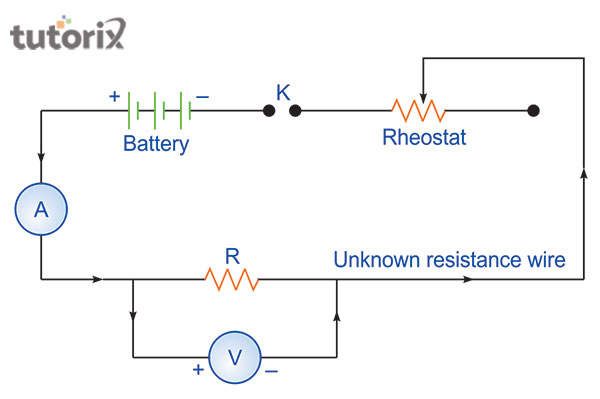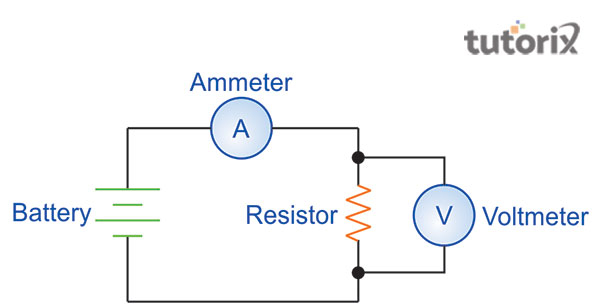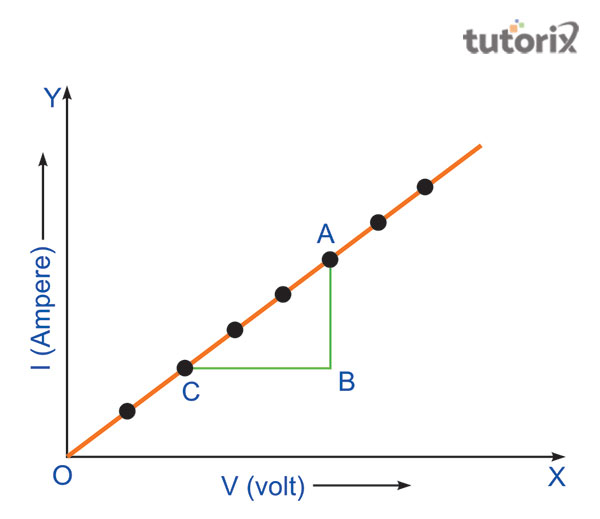# Determine Resistance Plotting Graph Potential Difference versus Current

## Introduction

Electrical circuit is referred to as the pathway that is associated with the transmission of the electric current. However, in this electrical circuit there lies a device that provides current to the charged particles. These charge particles constitute the current, for example, a battery or generator. The electrical circuit includes several devices such as, resistors, batteries, inductors, capacitors, and transistors or switches. However, the electrical circuit is a closed-loop that supports the flow of electrons through the wire.

## Theory and Circuit diagram

Ohm’s Law is referred to as the relation between the current flowing through the circuit and that of the current and resistance present within the circuit. In simple words, in this law, it is stated that the voltage or potential difference that is noted between the two points of the circuit is directly proportional to the current within the circuit (Yang, X., & Rogach, 2019). The law also states that the resistance is directly proportional to the voltage within the circuit. The expression is denoted by, V = I*R, where, V is the potential difference ,'' I  refers to current and R is the resistance. This further states, V $\propto$ I, that results in V = IR, where R is known as the proportionality constant.

Therefore, the relationship between the resistance of the material with the length and cross-sectional areas is expressed by, R = ρ(l/A), where, ρ is determined as the specific resistivity.Figure 1: Circuit diagram

In this practical experiment, the resistance is determined that is experienced with the closed electrical circuit. This experiment is to verify ohm's law and is conducted by plotting the recorded reading that is collected within this experiment, represented as the voltage vs current graph.Figure 2 : Schematic diagram of Ohm’s Law

## Aims and apparatus required

The aim of this practical experiment is to determine the resistance that is experienced due to the changes in the flow of current within the wire of the closed circuit. In this experiment, several apparatus are used, that include, wire of unknown resistance, voltammeter, plug key, sandpaper pieces'', connecting wires, rheostat, milliammeter and lastly, a battery (Dörfler et al. 2018). However, the electrical circuit needs to be checked properly before starting the experiment.

## Lab Procedures

The first step relates to the arrangement of the circuit as stated in figure 1, with proper cleaning of the ends of the wire that are connecting each other. The insulations are removed with the help of sandpaper. At the time of generating connections, the positive terminals of the ammeter and voltammeter are joined with the positive ends of the battery. Determination of the least count is noted and as well the possible errors are to be noted. After this, the key (K) is inserted and observation is conducted.

## Observations, calculations and results

Observations are recorded based on noting down the length, of the resistance wire followed by the range of both the given voltammeter and ammeter. Least count, zero error and zero correction are also noted for the voltmeter and ammeter as shown in the table below. In calculations, the graph is plotted for current and potential differences and as a result, resistance of the wire is calculated based on the ohm per centimetre-1.

No. of observations Applied potential difference in voltameter (V) Current flowing through the wire in milliammeter (A)

Figure 3: Table for observation current and voltageFigure 4: Graph between the potential difference across the wire V and the current I flowing through the wire

## Precautionary measures

The first measure is to check whether the connections within the electrical circuit are done neatly and it is clean and tightly done. Copper wires are to be used for connecting purposes that are thick in nature. Sandpaper is to be used for rubbing the insulation that is in the ends of the copper wires (Boardsolution, 2022). Voltameter and ammeter having proper ranges are to be used with a low resistance of rheostat and the key needs to be only used when the observations are taken.

## Sources for errors

Few sources of errors are there, such as the loosening of the instrument screws. The next is the availability of the thick connecting wires and lastly, rheostat that may have high resistance.

## Conclusion

In this tutorial, the practical experiment for determining the resistance that is experienced within the electrical circuit has been focused on. The verification of Ohm’s Law is conducted through this experiment. It is understood that the voltage is directly proportional to the current flowing and resistance experienced within the circuit. However, certain precautionary measures are to be kept in mind.

## FAQs

Q1. What is Ohm’s Law and its mathematical expression?

Ans. Ohm’s law is defined as the law of electricity, where the strength is associated with the direct current and tends to be directly proportional with the differences in potencies. In addition to these, the strength of current is inversely proportional to the resistance present within the circuit. The mathematical expression of Ohm’s Law is, I = E/R, where, I is denoted as current expressed in amps, E refers to voltage expressed in volts and lastly, R determines the resistance expressed in ohms.

Q2. What are the factors that influence the resistance of a conductor?

Ans. There lie many factors that affect the resistance of a conductor, that includes, resistivity experienced within the circuit, length of the wire or circuit, temperature and lastly, the cross-sectional area of the wire within the circuit.

Q3. What is electrical potential?

Ans. Electrical potential in the field of physics is defined as the amount of work that is necessary to displace one unit charge. This displacement occurs from the point of reference to any particular point against the electric field. It needs to be noted that the reference point is earth.